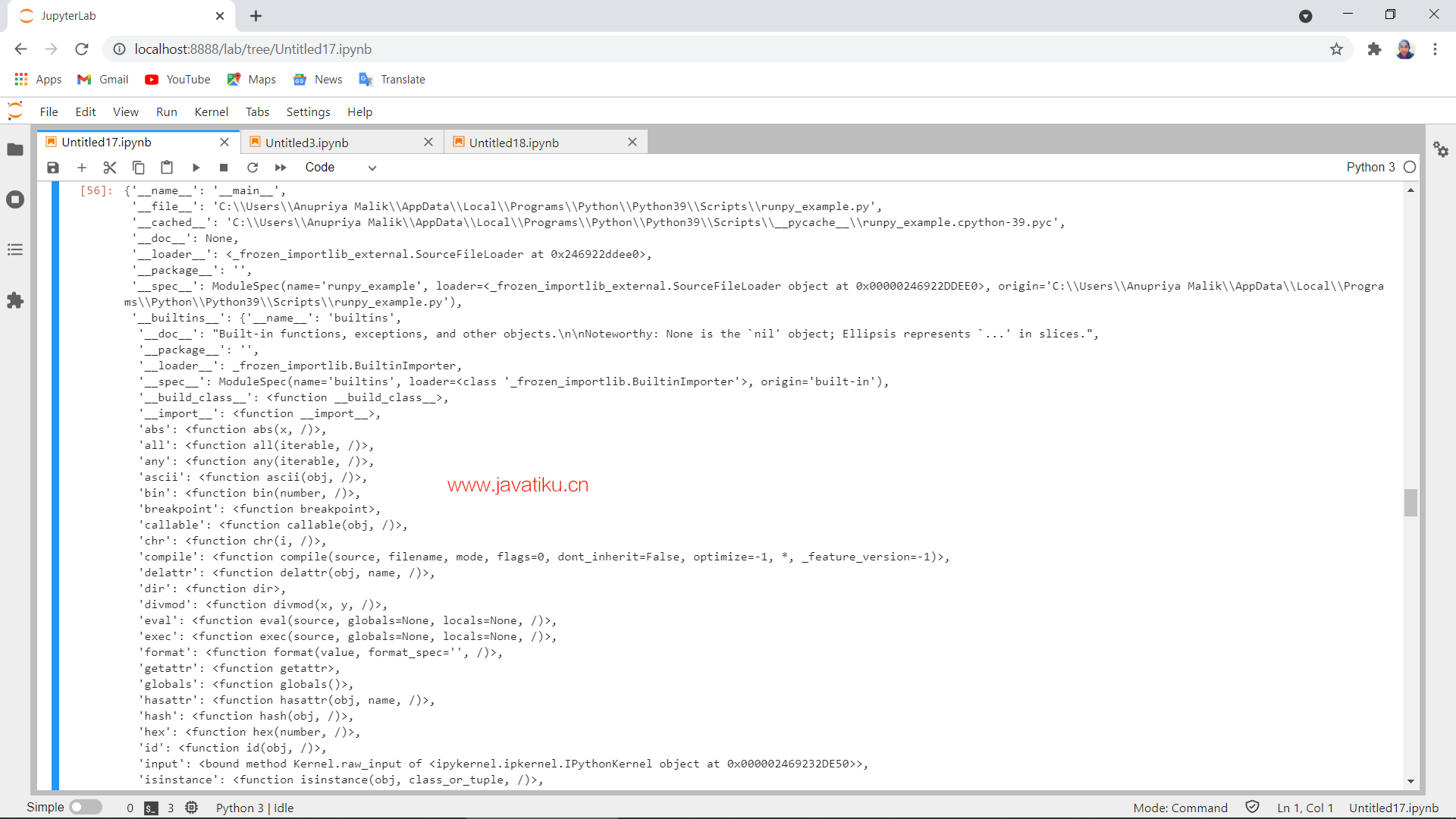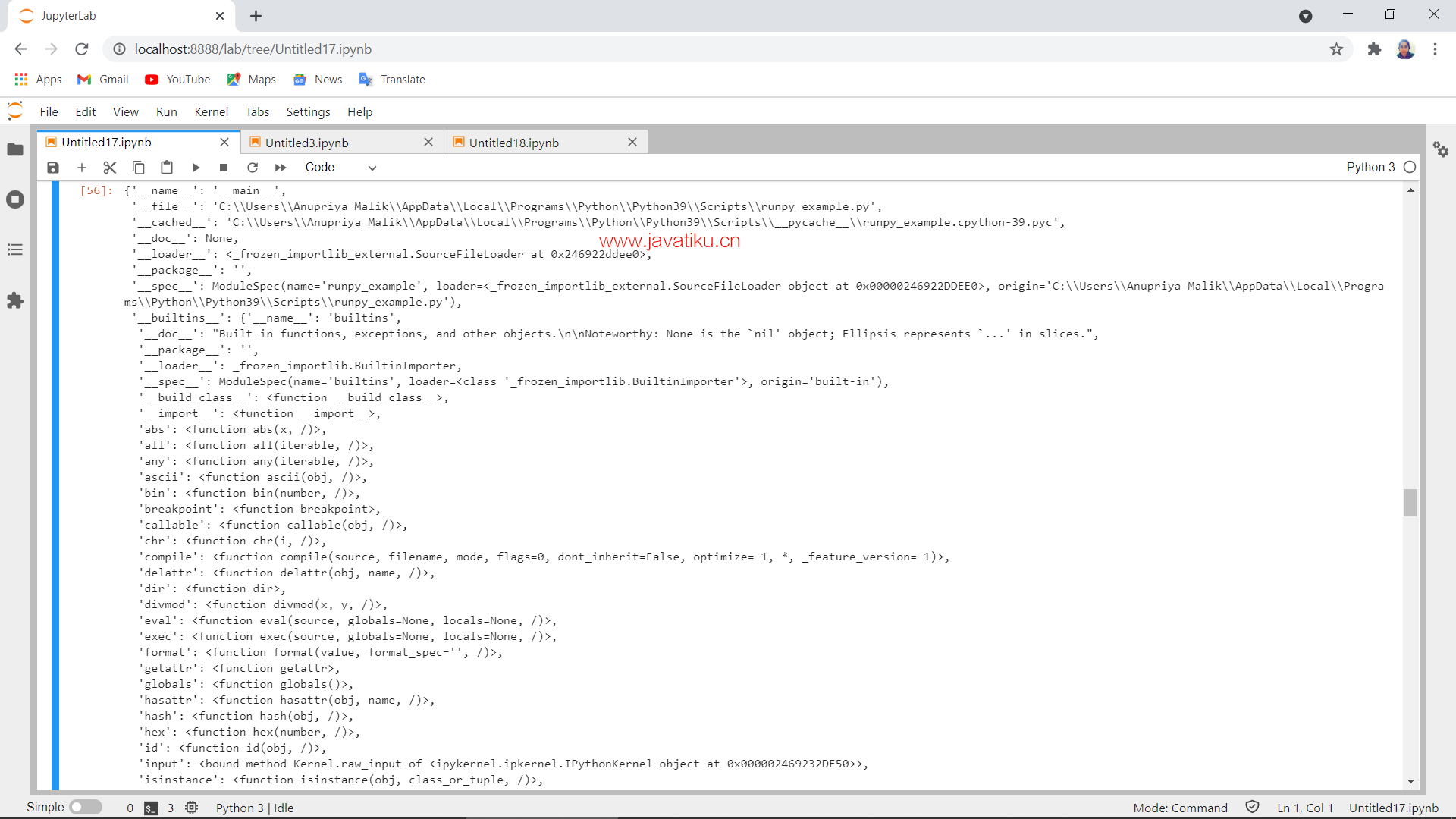# Python教程-在Python中查找和执行模块## PYTHONPATH变量

PYTHONPATH变量是一个基于平台的变量，由目录列表组成。其语法与shell变量的PATH相同。

``set PYTHONPATH = c: \\python3\\lib; ``

``set PYTHONPATH = /usr/local/lib/python  ``

## 在Python中执行模块

runpy模块定义了两个函数：

1.run_module()

2.run_path()

### run_module()

run_module()函数用于执行包含特定模块的代码，并返回模块全局字典的结果。

module_name参数应该是实际的模块名称。如果模块名称引用了任何包而不是普通模块，则将导入该包，并执行包内的__main__子模块，然后返回模块全局字典的结果。

### run_path()

run_path()函数用于执行给定路径上文件中的程序，并将模块全局字典作为结果返回。给定的路径可以是Python源文件、已编译的字节码文件或包含__main__模块的有效sys.path条目，例如包括顶级__main__.py文件的zipfile。

``````def add(p, q, r, s, t):
return p + q + r + s + t
def main():
p = 4
q = 6
r = 2
s = 8
t = 7
print ("sum of p, q, r, s, t = ")
return
if __name__=='__main__':
main()  ``````

``````import runpy_example as runp
runp.main()  ``````

``````sum of p, q, r, s, t =
27``````

``````import runpy
runpy.run_module('runpy_example', run_name='__main__')  ``````

``````sum of p, q, r, s, t =
27````````runpy.run_path('runpy_example.py', run_name='__main__')  ``

``````sum of p, q, r, s, t =
27````````C:\python37>python -m runpy_example  ``